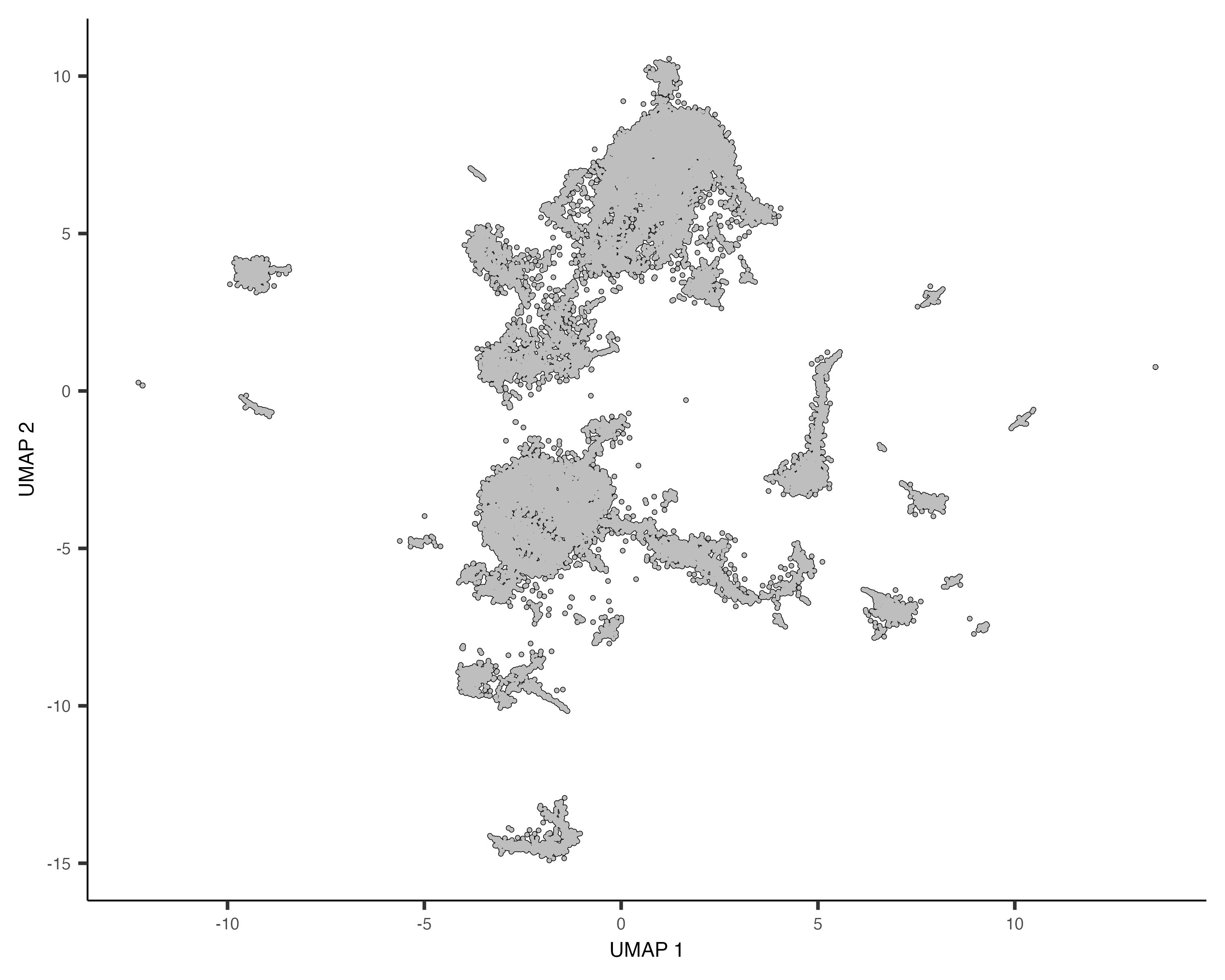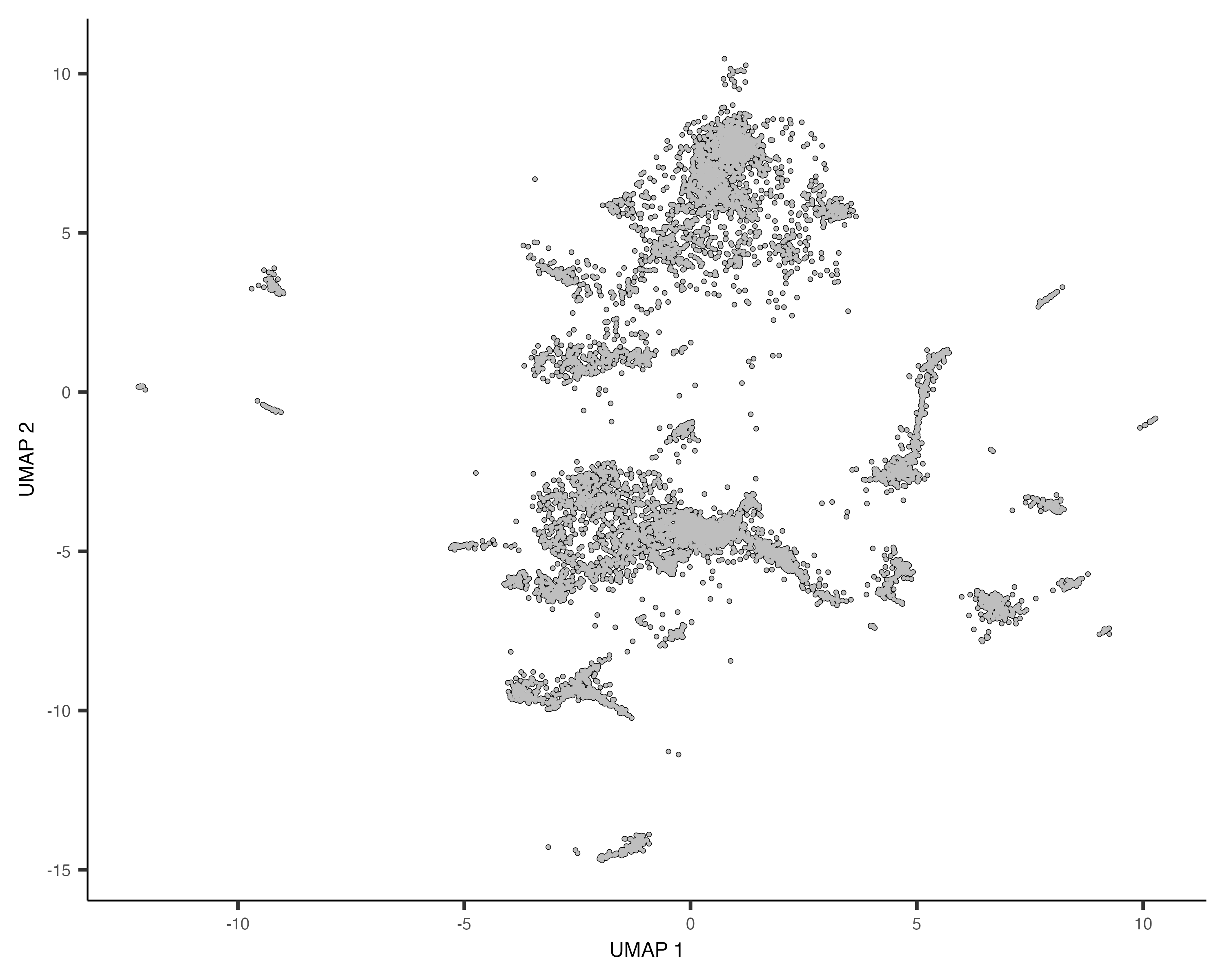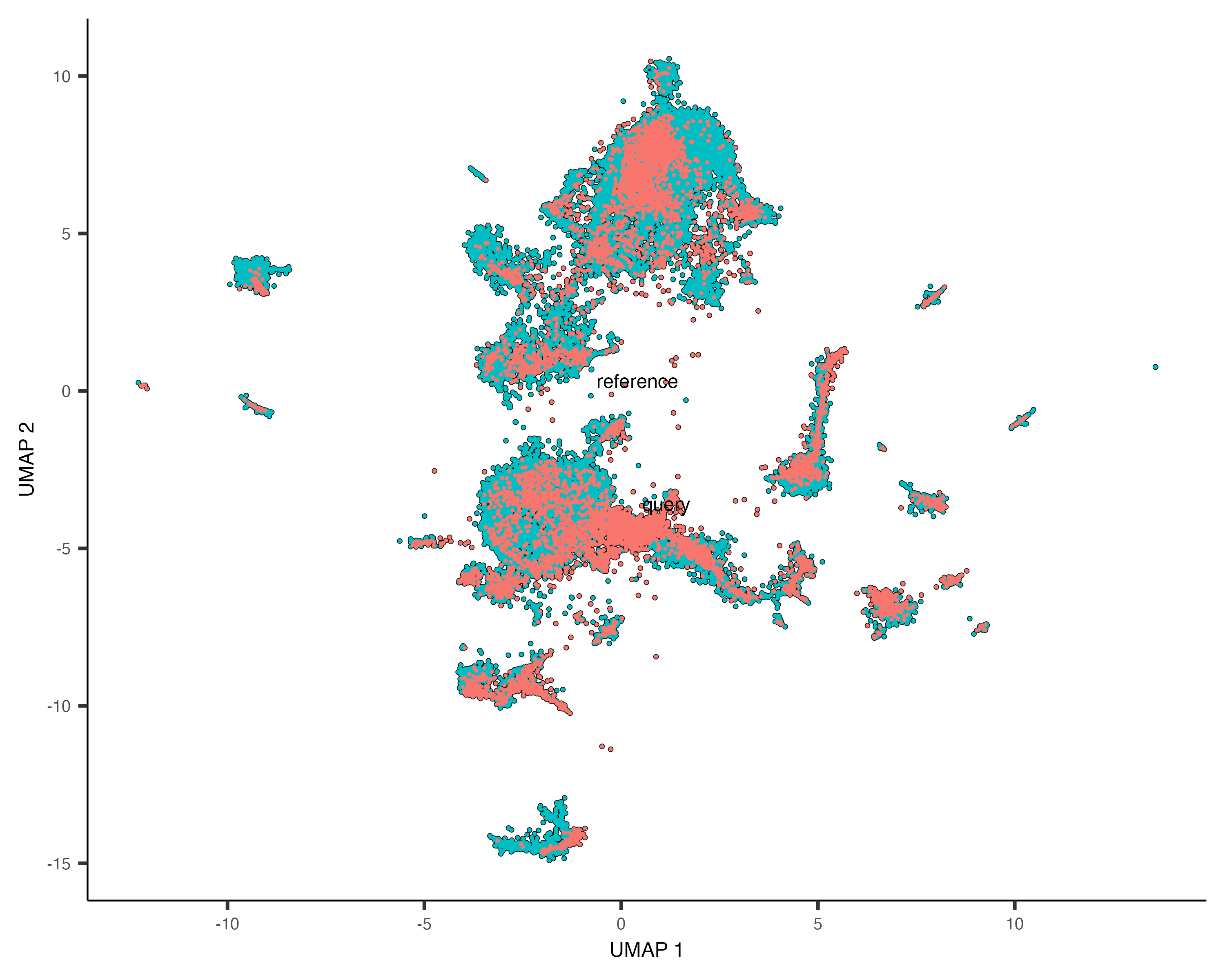## Project a query data set onto a reference data set

Co-embedding is used to compare similar data sets in order to identify similarities and differences and transfer annotations between cells. When the data sets are large and there are many sets to compare, the memory and run time requirements for processing co-embedded data can become impediments. Monocle3's solution is to save the models that transform a reference data set to low-dimensional PCA and UMAP space, and then use the models to transform query data sets to the low-dimensional reference space where one can plot them for comparison or annotate query cells by finding similar reference cells.

### Load the reference and query data sets

We begin by loading the reference and query data sets into Monocle3. To simplify this example, we load both data sets together; however, one can process them independently.

### Remove genes that are not in both data sets

It is essential that the query cds has the same genes in the same order as the reference cds, so we identify the shared genes and sort them.

### Apply a common UMI cutoff

We filter the cells by applying the same UMI cutoff to our data sets. For these data sets, we applied a cutoff of 1000 to reduce their sizes but we show how to find these cutoffs.

### Estimate size factors

After applying the gene and UMI filters, we re-calculate the size factors of both data sets.

### Process the reference data set

We are ready to process the reference data set by transforming it into PCA and and UMAP low dimension spaces. By setting the build_nn_index to TRUE, we build a nearest neighbor index in the UMAP space, which we will use to transfer the reference annotations to the query. After processing, we save the transform models and nearest neighbor index so that we can use them to transform the query data set into the reference space.

### Project the query data set into the reference space

Our query data set was loaded and filtered already, so we can use the preprocess_transform() and reduce_dimension_transform() functions to transform the query into the reference space. The load_transform_models() function loads the reference transform models into the query cds where they are used by preprocess_transform() and reduce_dimension_transform().

### Plot the combined data sets

First we plot the reference and query cells in UMAP space.### Plot the combined data sets

Now we label the cells in the reference and query cdses, combine the cdses, and plot the combined cells.### Transfer the reference cell labels to the query data set

Now we transfer the cell type annotations from the reference to the query data set using the nearest neighbor index that we made in the reference UMAP space.